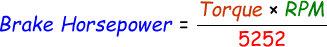# Brake Horsepower Calculator

Brake Horsepower, also in short known as BHP is the amount of work by done by an engine and how fast the engine could work. In other words, we can say it is the amount of watts generated at the engine's crankshaft. If the engine generates 746 watts then it means 1 horsepower.

Formula to calculate break horsepower is:In our below online brake horsepower calculator, enter the torque and RPM in the input boxes and click calculate to find the result.

 Torque: RPM: Brake Horsepower:

Latest Calculator Release

Average Acceleration Calculator

Average acceleration is the object's change in speed for a specific given time period. ...

Free Fall Calculator

When an object falls into the ground due to planet's own gravitational force is known a...

Torque Calculator

Torque is nothing but a rotational force. In other words, the amount of force applied t...

Average Force Calculator

Average force can be explained as the amount of force exerted by the body moving at giv...

Angular Displacement Calculator

Angular displacement is the angle at which an object moves on a circular path. It is de...